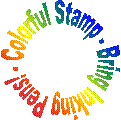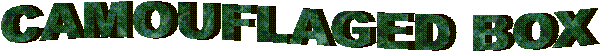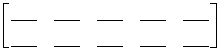Mathman 29 Just Balls Letterbox by Andrea Henning, Colton Berger, Gregg Rathke, Doug MartinTerrain: Grassy park and sidewalks Difficulty: Depends on math ability Placed by: The Dragon (Mathman) and the Honors Advanced Algebra students and teacher of 2007-08 Location: Jackson, WI County: Washington, WI Materials needed: Calculator, Compass, Writing Utensil, Personal Stamp, Inking PensStudents and parents of students should read my introduction to letterboxing before seeking the boxes.

Be sure to read through all the clues before looking for the boxes so you know what is expected of you.

Clues:

1. Start in the parking lot across from the restaurant MiMi’s in Jackson.

2. Turn and face MiMi’s. Write down the year that the building was created. (Hint- It should be towards the top)

3. Add the digits of the year up to make the number x = _____ and use the following equation to find out how many paces you should take towards the Jackson Mill along the sidewalk.

steps = 9x(1/3)=______

4. On your right you will find Reynolds Avenue. Go to the beginning of the sidewalk and use this equation to find how many paces you should take down Reynolds:

x2 - 29x + 138 = 0

Find the roots and multiply their absolute values together, then multiply by two: ________

5. You should be at a red octagon across from a beige house.
Look at the beige house’s address and add the digits up (a = ______). Solve x - 20 = 2a   The solution will be the number of paces you should take on the following heading.

6. Use the equation x3=729,000. This gives you the number of degrees you should turn counter-clockwise when facing the red octagon.  Now take your steps.

7. At this intersection, you should see a speed limit sign to the left. Square the number of sides on the sign, and your answer will represent x.

8. Now look south again and count the number of fire hydrants you see down the road. This represents y.

9. Use the equation x/y to find the total number of mailboxes/newspaper boxes straight ahead of you along the road. Walk to the 3-way intersection.

10. Solve the equation x2 = 8100. This gives your next bearing.

11. Using the word MEADOWVIEW, assign each letter a number 1-26 as it corresponds in the alphabet. Use these numbers to create 5x2 Matrix A.12. Do the same thing with the word PARK. Assign these numbers to a 2x2 matrix B.13. Multiply your matrices together to form Matrix C. Subtract the bottom row of Matrix C from the top row, and subtract 100 from your answer. Take that many steps east on the sidewalk to arrive at a fun zone for little children.

14. Walk to the “fine sign” and admire the beauty of the playground.

15. Directly behind the sign is a yellow “bucket” used for a game. Add the numbers located on it, representing Y = ________

16. Locate the emergency vehicle in the playground, and multiply the numbers on the back right of the car to find E = _______

17. Use the equation (Y)(E) = x to find the next bearing. (Hint- x is your bearing.)

18. Execute your bearing from where you were in step 14, and walk to your destination to get a well-deserved rest.

19. Look high and low while you do a little exploring to find your treasure.

BEFORE RETRIEVING THE BOX, BE SURE NO ONE IS AROUND TO SEE YOU TAKE THE BOX OUT!  DO NOT BRING ATTENTION TO YOURSELF OR THE HIDING LOCATION OF THE BOX!

Good luck!

Before you set out read the waiver of responsibility and disclaimer.

LbNA Home